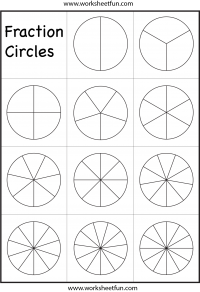Fraction Circles Template – Printable Fraction Circles – 1 WorksheetFraction Circles Template – Printable Fraction Circles

Halves, Thirds, Fourths, Fifths, Sixths, Sevenths, Eights, Ninths, Tenths, Elevenths, Twelfths

More fraction Circles Worksheets

Fraction

Fraction Circles

Fraction Circles Template

Fraction Model

Fraction Subtraction

Fractions – Coloring

Fractions – Comparing

Fractions – Equivalent